Courses

# Notes | EduRev

## NEET : Notes | EduRev

The document Notes | EduRev is a part of the NEET Course Physics 28 Years Past year papers for NEET/AIPMT Class 11.
All you need of NEET at this link: NEET

Q 1. A copper rod of 88 cm and an aluminium rod of unknown length have their increase in length independent of increase in temperature. The length of aluminium rod is:
(αCu = 1.7 × 10-5 K-1 and αAl = 2.2 × 10-5 K-1)    
A: 6.8 cm
B: 113.9 cm
C: 88 cm
D: 68 cm
Ans:
D
Solution:
αCuLCu = αAlLAl
1.7 × 10–5 × 88 cm = 2.2 × 10-5 × LAl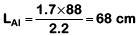Q 2. The power radiated by a black body is P and it radiates maximum energy at wavelength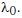If the temperature of the black body is now changed so that it radiates maximum energy at wavelength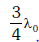, the power radiated by it becomes nP. The value of n is:-    
A: 3/4
B: 4/3
C: 256/81
D: 81/256
Ans: C
Solution: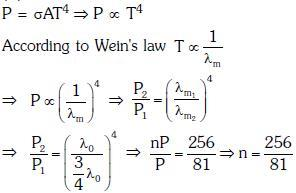Q 3. A spherical black body with a radius of 12 cm radiates 450 watt power at 500 K. If the radius were halved and the temperature doubled, the power radiated in watt would be:-    
A: 450
B: 1000
C: 1800
D: 225
Ans: C
Solution: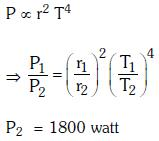Q 4. Two rods A and B of different materials are welded together as shown in figure. Their thermal conductivities are K1 and K2. The thermal conductivity of the composite rod will be:-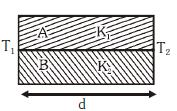A: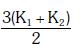B: K1 + K2
C: 2(K1 + K2)
D: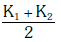Ans: D
Solution: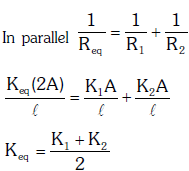Q 5. Coefficient of linear expansion of brass and steel rods are α1 and α2 . Lengths of brass and steel rods are ℓ1 and ℓ2 respectively. If (ℓ2 - ℓ1 ) is maintained same at all temperatures, which one of the following relations holds good ?    
A: α11 = α22
B: α12 = α21
C: α122 = α212
D: α122 = α221
Ans:
A
Solution:
Coefficient of linear expansion of brass = α1
Coefficient of linear expansion = α2
Length of brass and steel rods are l1 and l2 respectively.
Given,
Increase in length (l2'-l1' ) is same for all temperature.
So,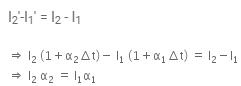Q 6. A piece of ice falls from a height h so that it melts completely. Only one-quarter of the heat produced is absorbed by the ice and all energy of ice gets converted in to heat during its fall. The value of h is : [Latent heat of ice is 3.4 x 105 J/Kg and g = 10 N/kg]    
A: 68 km
B: 34 km
C: 544 km
D: 136 km
Ans: D

Solution:
As per conservation of energy, energy gained by the ice during its fall from height h is given by, E = mgh,
Given, only one-quarter of its energy is absorbed by the ice.
So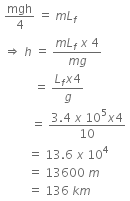Q 7. A block body is at a temperature of 5760 K. The energy of radiation emitted by the body at wavelength 250 nm is U1 at wavelength 500 nm is U2 and that at 1000 nm is U3. Wien's constant, b = 2.88 x 106 nmK. Which of the following is correct?     
A: U1 > U2
B: U1 = 0
C: U3 = 0
D: U2 > U1
Ans:
D
Solution:
Given, temperature, T1 = 5760 K
Given that energy of radiation emitted by the body at wavelength 250 nm in U1, at wavelength 500 nm is U2 and that at 1000 nm is U3.
Now, according to Wein's law, we get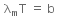where, b = Wien's constant = 2.88 x 106 nmK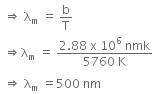λm is the wavelength corresponding to maximum energy, so U2 > U1.
Given, temperature, T1 = 5760 K
Given that energy of radiation emitted by the body at wavelength 250 nm in U1, at wavelength 500 nm is U2 and that at 1000 nm is U3.
Now, according to Wein's law, we get
λmT =b
where, b = Wien's constant = 2.88 x 106 nmK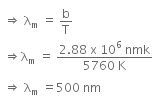λm is the wavelength corresponding to maximum energy, so U2 > U1.

Q 8. The two ends of a metal rod are maintained at temperatures 100oC and 110oC. the rate of heat flow in the rod is found to be 4.0 J/s. If the ends are maintained at temperatures 200oC and 210oC, the rate of heat flow will be:    
A: 4.0 J/s
B: 44.0 J/s
C: 16.8 J/s
D: 8.0 J/s
Ans:
A
Solution:
Here, ΔT1 = 110-100 = 10o C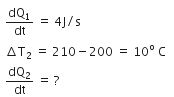As the rate of heat flow is directly proportional to the temperature difference and the temperature difference in both the cases is same i.e. 10o C. So, the same rate of heat will flow in the second case.
Hence,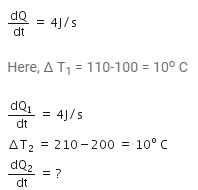As the rate of heat flow is directly proportional to the temperature difference and the temperature difference in both the cases is same i.e. 10o C. So, the same rate of heat will flow in the second case.
Hence,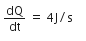Q 9. On observing light from three different stars P, Q and R, it was found that intensity of violet colour is maximum in the spectrum of P, the intensity of green colour is maximum in the spectrum of R and the intensity of red colour is maximum in the spectrum of Q. If TP, TQ and TR are the respective absolute temperatures of P, Q and R, then it can be concluded from the above observations that:    
A: TP < TQ < TR
B: TP > TQ > TR
C: TP > TR > TQ
D: TP < TR < TQ
Ans: B
Solution
: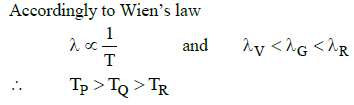Q 10. Light with an energy flux of 25 x 104Wm−2 falls on a perfectly reflecting surface at normal incidence. If the surface area is 15 cm2, the average force exerted on the surface is    
A: 1.20 x 10−6 N
B: 3.0 x 10−6 N
C: 1.25 x 10−6 N
D: 2.50 x 10−6 N
Ans:
D
Solution: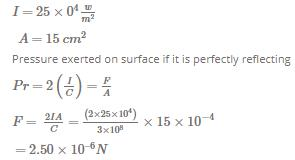Q 11. Certain quantity of water cools from 70°C to 60°C in the first 5 minutes and to 54° C in the next 5 minutes. The temperature of the surroundings is    
A: 42°C
B: 10°C
C: 45°C
D: 20°C
Ans:
C
Solution: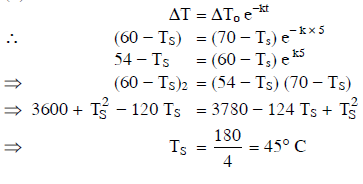Q 12. Steam at 100°C is passed into 20 g of water at 10°C. When water acquires a temperature of 80°C, the mass of water present will be:
[Take specific heat of water = 1 cal g−1 °C−1 and latent heat of steam = 540 cal g−1]    
A: 42.5 g
B: 22.5 g
C: 24 g
D: 31.5 g
Ans
: B
Solution: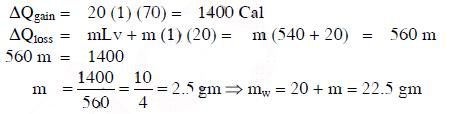Offer running on EduRev: Apply code STAYHOME200 to get INR 200 off on our premium plan EduRev Infinity!

14 docs|25 tests

,

,

,

,

,

,

,

,

,

,

,

,

,

,

,

,

,

,

,

,

,

;